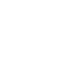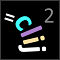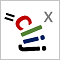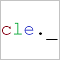# CLIJ2GPU accelerated image processing for everyone

CLIJ2 home

## averageDistanceOfTouchingNeighborsTakes a touch matrix and a distance matrix to determine the average distance of touching neighbors for every object.

### Parameters

distance_matrix : Image The a distance matrix to be processed. touch_matrix : Image The binary touch matrix describing which distances should be taken into account. distance_list_destination : Image A vector image with the same width as the distance matrix and height=1, depth=1. Determined average distances will be written into this vector.

Categories: Graphs, Measurements

Availability: Available in Fiji by activating the update sites clij and clij2. This function is part of clij2_-2.5.0.1.jar.

### Usage in ImageJ macro

``````Ext.CLIJ2_averageDistanceOfTouchingNeighbors(Image distance_matrix, Image touch_matrix, Image average_distancelist_destination);
``````

### Usage in object oriented programming languages

Java
```// init CLIJ and GPU
import net.haesleinhuepf.clij2.CLIJ2;
import net.haesleinhuepf.clij.clearcl.ClearCLBuffer;
CLIJ2 clij2 = CLIJ2.getInstance();

// get input parameters
ClearCLBuffer distance_matrix = clij2.push(distance_matrixImagePlus);
ClearCLBuffer touch_matrix = clij2.push(touch_matrixImagePlus);
average_distancelist_destination = clij2.create(distance_matrix);
```
```// Execute operation on GPU
clij2.averageDistanceOfTouchingNeighbors(distance_matrix, touch_matrix, average_distancelist_destination);
```
```// show result
average_distancelist_destinationImagePlus = clij2.pull(average_distancelist_destination);
average_distancelist_destinationImagePlus.show();

// cleanup memory on GPU
clij2.release(distance_matrix);
clij2.release(touch_matrix);
clij2.release(average_distancelist_destination);
```
Matlab
```% init CLIJ and GPU
clij2 = init_clatlab();

% get input parameters
distance_matrix = clij2.pushMat(distance_matrix_matrix);
touch_matrix = clij2.pushMat(touch_matrix_matrix);
average_distancelist_destination = clij2.create(distance_matrix);
```
```% Execute operation on GPU
clij2.averageDistanceOfTouchingNeighbors(distance_matrix, touch_matrix, average_distancelist_destination);
```
```% show result
average_distancelist_destination = clij2.pullMat(average_distancelist_destination)

% cleanup memory on GPU
clij2.release(distance_matrix);
clij2.release(touch_matrix);
clij2.release(average_distancelist_destination);
```
Icy JavaScript
```// init CLIJ and GPU
importClass(net.haesleinhuepf.clicy.CLICY);
importClass(Packages.icy.main.Icy);

clij2 = CLICY.getInstance();

// get input parameters
distance_matrix_sequence = getSequence();
distance_matrix = clij2.pushSequence(distance_matrix_sequence);
touch_matrix_sequence = getSequence();
touch_matrix = clij2.pushSequence(touch_matrix_sequence);
average_distancelist_destination = clij2.create(distance_matrix);
```
```// Execute operation on GPU
clij2.averageDistanceOfTouchingNeighbors(distance_matrix, touch_matrix, average_distancelist_destination);
```
```// show result
average_distancelist_destination_sequence = clij2.pullSequence(average_distancelist_destination)
// cleanup memory on GPU
clij2.release(distance_matrix);
clij2.release(touch_matrix);
clij2.release(average_distancelist_destination);
```
clEsperanto Python (experimental)
```import pyclesperanto_prototype as cle

cle.average_distance_of_touching_neighbors(distance_matrix, touch_matrix, average_distancelist_destination)

```

Imprint